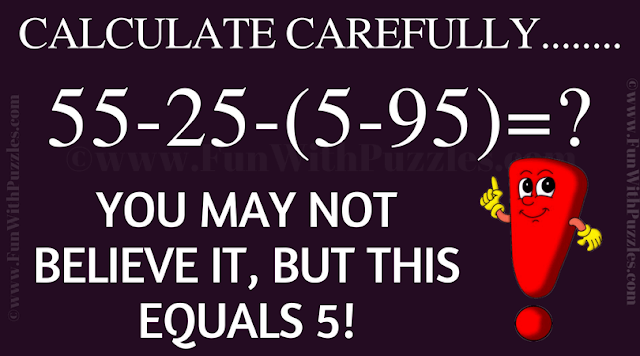This Brain Teaser is to test your Mathematical Skills. In this Math Brain Teaser, there is one Arithmetic Equation. You have to calculate the value of this Maths Equation. Once you calculate this equation, you will be surprised by the answer. Do you agree with the answer given the puzzle image?Brain Teaser to test your Mathematical Skills